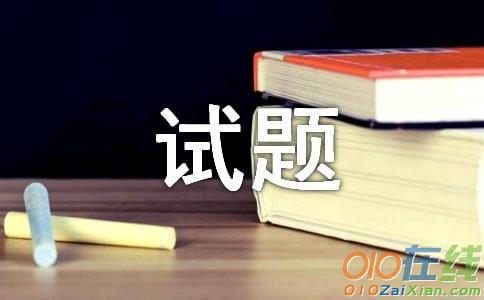# 小学四年级奥数试题及答案

导语：在学习、工作中，我们都不可避免地要接触到试题，试题是命题者按照一定的考核目的编写出来的。什么类型的试题才能有效帮助到我们呢？以下是小编收集整理的小学四年级奥数试题及答案，欢迎大家借鉴与参考，希望对大家有所帮助。## 小学四年级奥数试题及答案篇1

_____年级 _____班 姓名_____ 得分_____

1. 加工一批39600件的大衣,30个人10天完成了13200件,其余的要求在15天内完成,要增加_____人.

2. 54人12天修水渠1944米,如果人数增加18人,天数缩到原来的一半,可修水渠_____米.

3. 一批产品,28人25天可以收割完,生产5天后,此项任务要提前10天完成,应增加_____人.

4. 某食堂存有16人可吃15天的米,16人吃了5天后,走了6人,余下的可吃_____天.

5. 某生产小组12个人,9天完成,零件1620个.现在有一批任务,零件数为2520个,问14个人要_____天完成.

6. 一项工程预计15人每天做4小时,18天可以完成,后来增加3人,并且工作时间增加1小时,这项工程_____天完成.

7. 某机床厂第一车间的职工,用18台车床,2小时生产机器零件720件,20台这样的车床3小时可生产机器零件_____件.

8. 4辆大卡车5次运煤80吨,3辆小卡车8次运煤36吨.现在有煤77吨,用一辆大卡车和小卡车同时运_____次运完.

9. 某车间接到任务,要在15天制造12000个零件.后来任务增加28%日产量也提高 .这样_____天完成.

10. 8个人10天修路840米,照这样算,20人修4200米,要_____天.

解答题:

11. 某工程队施工时,欲将一个池塘的水排完,若用15台抽水机,并且每天抽水8小时,则7日可排水1260吨;若每天抽水12小时,要求14天排水7560吨,则应需几台抽水机?

12. 光华机械厂一个车间,原计划15人3天做900个零件,生产开始后,又增加一批任务,在工作效率相同下,要10个人8天完成,问增加了几个零件?

13. 光明小学有50个学生帮学校搬砖,要搬2000块,4次搬了一半,照这样算,再增加50个学生,还要几次运完?

14. 一根木料,锯成2段,要3分钟,如果锯成6段要多少分钟?

---------------答 案----------------------

1. 10人.

解: (39600-13200)÷(13200÷30÷10×15)-30=10(人).

2. 1296米.

解: 1944÷54÷12×(18+54)×(12÷2)=1296(米).

3. 28人.

解: (28×25-28×5)÷(25-5-10)-28=28(人).

4. 16天.

解: (15×16-5×16)÷(16-6)=16(天).

5. 12天.

解: 2520÷(1620÷9÷12×14)=12(天).

6. 12天.

解: 15×4×18÷[(15+3)×(4+1)]=12(天).

7. 1200件.

解: 720÷18÷2×20×3=1200(件).

8. 14次.

解: 77÷[(80÷4÷5)+(36÷3÷8)]=14(次).

9. 16天.

解: (12000+12000×0.28)÷(12000÷15+12000÷15× )=16(天).

10. 20天.

解: 4200÷(840÷10÷8×20)=20(天).

11. 先求出1台机器1小时排水的吨数: 1260÷7÷8÷15=1.5(吨).

再求出1台机器每天排12小时排足14天的水的吨数: 1.5×12×14=252(吨).

最后求出所需要的台数: 7560÷252=30(台).

综合式: 7560÷[1260÷15÷(8×7)×(12×14)]=30(台).

12. 先求出每个人每天做的个数: 900÷15÷3=20(个).

再求出共做的个数: 20×10×8=1600(个).

最后求出增加的个数: 1600-900=700(个).

13. 先求出每个学生每次运的砖数: 2000× ÷4÷50=5(块).

再求出现在的学生一次过运的砖数: (50+50)×5=500(块).

最后求出还要运的次数: 2000× ÷500=2(次).

简便方法: 4÷[(50+50)÷50]=2(次).

14. 先求出锯一下用的时间: 3÷(2-1)=1.5(分钟).

再求出锯6段用的次数: 6-1=5(次).

最后求出共用的时间: 1.5×5=7.5(分钟).

## 小学四年级奥数试题及答案篇2

1. 某校安排学生宿舍,如果每间5人,则有14人没有床位;如果每间7人,则多4个床位.该校有宿舍_____间,学生_____人.

2. 用库存化肥给麦田施肥,如果每公亩施6千克,就缺200千克;如果每公亩施5千克,则剩下300千克,那么有_____公亩麦田,库存化肥_____千克.

3. 用一根绳子测量井的深度,如果线绳两折时,多5米,;如果绳子3折时,差4米,绳子长_____米,井深_____米.

4. 小玲买5千克苹果,可多余1元8角钱;如果买6千克,还差1元2角.每千克苹果价钱是_____元,小玲带的钱是_____元.

5. 某校学生参加劳动,分成若干组,如果10人一组,正好分完,如果12人一组,差10人.参加劳动的有_____人.

6. 挖一条水渠,如果每人挖24米,则超过总长120米,如果每人挖30米,则超过总长300米.挖渠共有_____人,渠长_____米.

7. 一根绳子,如果剪5段,则差2米;如果剪3段,则余下8米.绳子长_____米.

8. 箱子里有若干只袜子,如果每次取7只,则剩下6只,如果每次取9只,则差8只.箱子里_____只袜子.

9. 工人铺一条路基,若每天铺260米,铺完全路长就得延长8天;若每天铺300米,铺完全路长仍要延长4天,这条路长_____米.

10. 一堆桃子分给一群猴子,如果每只猴子分10个桃子,则有两只猴没有分到,如果每只猴子分8个,则刚好分完.有_____个桃子.

解答题:

11. 幼儿园有梨数是桃子数的2倍,分给幼儿园小朋友,每人分桃5个,最后余下15个;每人分梨14个,则梨数差30个.问幼儿园有桃、梨多少个?

12. 课外活动跳绳比赛,其中2组各借绳4根,其余的组借5根,这样分配最后余下12根;如果每组借6根,这样恰好借完.问有绳多少根?

13. 小明用一元买了5支铅笔和8块橡皮,余下的.钱,如果买一支铅笔就不足2分;如果买一块橡皮就多出1分.每支铅笔多少分?每块橡皮多少分?

14. 小玲从家去学校,如果每分钟走80米,结果比上课时间提前6分钟到校.如果每分钟走50米,则要迟到3分钟,小玲的家到学校有多远?

答 案:

1. 59人.

解: (14+4)÷(7-5)=9(间);

9×5+14=59(人).

2. 500公亩; 2800千克.

解: (300+200)÷(6-5)=500(公亩);

500×5+300=2800(千克).

3. 54米,22米.

解: (5×2+4×3)÷(3-2)=22(米);

(22-4)×3=54(米).

4. 16.8元.

解: (1.8+1.2)÷(6-5)=3(元);

3×5+1.8=16.8(元).

5. 50人.

解: 10÷(12-10)=5(组),5×10=50(人).

6. 30人; 600米.

解: (300-120)÷(30-24)=30(人);

30×30-300=600(米).

7. 23米.

解: (8+2)÷(5-3)×5-2=23(米).

8. 55只.

解: (6+8)÷(9-7)×9-8=55(只).

9. 7800米.

解: 260×8-300×4=880(米);

880÷(300-260)=22(天);

260×(22+8)=7800(米).

10. 80个.

解: (10×2)÷(10-8)=10(只),10×8=80(个).

11. 90个; 180个.

解: 因为梨数是桃数2倍,如果每人分梨5×2=10(个),最后余下15×2=30

(个).因为14个比5个的2倍多14-5×2=4(个),分到最后差30个.所以30+30=60

(个)为总差,每次多分4个为分差,幼儿园有60÷4=15(人).

桃数有5×15+15=90(个),梨有90×2=180(个).

12. 10组; 60根.

解: [12-(5-4)×2]÷(6-5)=10(组);

6×10=60(根).

13. 6分.

解: 如果小明多2分钱的话,正好可以买6支铅笔和8块橡皮.从总的钱数中减去铅笔比橡皮贵的钱,剩下的钱正好是14块橡皮的价钱,可用除法先求出每块橡皮的价钱,进而求出每支笔的价钱.

铅笔:6+2+1=9(分)

橡皮:[100+2-(2+1)×(5+1)]÷14=6(分).

14. 1200米.

解: (80×6+50×3)÷(80-50)=21(分),(21-6)×80=1200(米).

## 小学四年级奥数试题及答案篇3

地理老师在黑板上挂了一张世界地图,并给五大洲的每一个洲都标上一个代号,让学生认出五个洲,五个学生分别回答如下

甲:3号是欧洲,2号是美洲;

乙:4号是亚洲,2号是大洋洲;

丙:1号是亚洲,5号是非洲;

丁:4号是非洲,3号是大洋洲;

戊:2号是欧洲,5号是美洲。

老师说他们每人都只说对了一半,1号_______,2号_______,3号_______,4号________,5号_________。

答案与解析：1号是亚洲;2号是大洋洲;3号是欧洲;4号是非洲;5号是美洲。

苏教版小学四年级奥数题及答案《回答问题》：假设甲说的前半句是对的，则3号是欧洲，由此推出丁说的3号是大洋洲是错误的。由于每个人都只说对了一半，可知丁说的4号是非洲是对的，由此推出乙说的4号是亚洲是错的，2号是大洋洲是对的。又可知戊说的2号是欧洲是错的，5号是美洲是对的，由此推出丙说的5号是非洲是错的，1号是亚洲是对的，最后得到正确的结论是:1号是亚洲;2号是大洋洲;3号是欧洲;4号是非洲;5号是美洲。

## 小学四年级奥数试题及答案篇4

正方体盒子的每个面上都写有一个自然数，并且相对两个面所写的两数之和都相等.若18对面所写的是质数a;14对面所写的是质数b;35对面所写的质数是c.试求a+b+c的值.

考点：奇偶性问题;质数与合数问题.

分析：根据题目已知18+a=14+b=35+c.18和14是偶数，而35是奇数，除2之外所以的质数都是奇数，因为18+a和14+b的和肯定是奇数，所以35+c也只能是奇数，所以a，b肯定是奇质数，不会是唯一的偶质数2，那么c就只能是偶质数2了，知道c=2，也可以知道b=23，a=19.最后a+b+c=44.

解答：解：已知18+a=14+b=35+c.

a，b肯定是奇质数，不会是唯一的偶质数2，那么c就只能是偶质数2;

35+c=35+2=37;

18+a=37，

a=37-18=19;

14+b=37，

b=37-14=23;

a+b+c=19+23+2=44.

点评：根据质数的奇偶性的特点，以及奇数+偶数=奇数的特点，找出c是偶数质数2，再进一步求解.

【小学四年级奥数试题及答案】相关文章：

1.奥数试题及答案

2.奥数行程试题及答案

4.小升初奥数试题及答案(十)

5.初中奥数试题及答案

6.小学奥数试题及答案

7.小学奥数精选试题及答案

8.小升初奥数测试题及答案

9.小学奥数测试题及答案